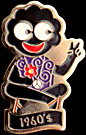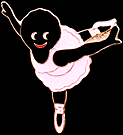## GOLLY SURVEY RESULTS – ANALYSIS 1

The following analysis was taken from 50 responses received over a period of 6 months to August 2001. Replies were solicited only by the survey's presence on this web site. No emails or off-line promotion was used to request responses.

The survey remains open. The results will be updated at significant milestones (e.g. 1 year or 100 replies). If anybody feels their previous answers may be out of date and would like to resubmit a survey form, I will ensure this supersedes their previous entry.

Thanks to those who have participated so far, both old friends and newcomers alike. If you have not yet taken part and would like to do so, it's not too late.

How many took part? 50

What was the gender split?
52% Male, 48% Female. Robertson's have found from their survey results that the majority of collectors are male. Going back to around 1996, most Internet users were also thought to be male. This result bucked both those trends showing a fairly even split.

Where are you from?
96% from within the UK. 2% were from the U.S. and 2% from Australia. Of those within the UK several took the trouble to state their regions which included: – Scotland, N. Ireland, Yorkshire, London and even Bishop Auckland!

How many have WAP enabled phones?
10%. I think the web site is going to be more popular than the WAP site for a while longer!

What is collected?
 Golly collection 10% 20% 30% 40% 50% Brooches X X X X X X X X X X X X X X X X X X X X Various X X X X X X X X X X X X X X X X X X X X All things Golly X X X X X X X X X X X X X X X X X X X X
Golly Corner specifically targets brooch collectors. Whether this result reflects this is the single most popular area of Golly Collecting, or whether it is because of the way this site targets those collectors has not been determined.
Were the participants regulars to the site?
 Frequency 10% 20% 30% 40% First time X X X X X X X X X X X X X X X X Every few months X X X X X X X X X X X X X X X X Each month X X X X X X X X X X X X X X X X Each Week X X X X X X X X X X X X X X X X More than once a week X X X X X X X X X X X X X X X X
Many first time visitors to the site took part in the survey, but over 70% of the responses were from visitors who regularly check the site.
When did the participants get Internet access?
 Year 10% 20% 35% 40% Blank X X X X X X X X X X X X X X X X 93/94 X X X X X X X X X X X X X X X X 95/96 X X X X X X X X X X X X X X X X 97/98 X X X X X X X X X X X X X X X X 99/00 X X X X X X X X X X X X X X X X 01/02 X X X X X X X X X X X X X X X X
The rate at which collectors have been getting on-line since 1997 appears exponential – this is confirmed by the site's hit counter which started in 1996. At some point this growth rate must slow down as those who are interested in Internet access will already be connected. Note that the final period shown – 2001/2002 is not yet complete as the survey only includes results to August 2001.
Year Collection Started
 Year 10% 20% 30% 40% 50% 1940s X X X X X X X X X X X X X X X X X X X X 1950s X X X X X X X X X X X X X X X X X X X X 1960s X X X X X X X X X X X X X X X X X X X X 1970s X X X X X X X X X X X X X X X X X X X X 1980s X X X X X X X X X X X X X X X X X X X X 1990s X X X X X X X X X X X X X X X X X X X X 2000s X X X X X X X X X X X X X X X X X X X X
The majority of responses are from collectors who started in the 1980s or later. Given those taking part are using the Internet which is still weighted towards younger users, this is not too surprising.
Size of Collection
 Size 10% 20% 30% 40% 50% 60% 70% Upto 100 X X X X X X X X X X X X X X X X X X X X X X X X X X X X 101 to 200 X X X X X X X X X X X X X X X X X X X X X X X X X X X X 201 to 300 X X X X X X X X X X X X X X X X X X X X X X X X X X X X 301 to 400 X X X X X X X X X X X X X X X X X X X X X X X X X X X X
Golly's Golden Era
 Era 5% 10% 15% 20% Pre War X X X X X X X X X X X X X X X X 1950s/60s X X X X X X X X X X X X X X X X 1970s X X X X X X X X X X X X X X X X 1980s X X X X X X X X X X X X X X X X 1990s X X X X X X X X X X X X X X X X Now X X X X X X X X X X X X X X X X Blank X X X X X X X X X X X X X X X X
Golly's Dark Era
 Era 5% 10% 15% 20% 25% 30% Pre War X X X X X X X X X X X X X X X X X X X X X X X X 1950s/60s X X X X X X X X X X X X X X X X X X X X 1970s X X X X X X X X X X X X X X X X X X X X X X X X 1980s X X X X X X X X X X X X X X X X X X X X X X X X 1990s X X X X X X X X X X X X X X X X X X X X Now X X X X X X X X X X X X X X X X X X X X X X X X Blank X X X X X X X X X X X X X X X X X X X X X X X X
Pre-war and the current eras are popular. The 1950s/1960s and early 1990s less so. 30% of people could not nominate a 'dark era for Golly'. This reluctance to be negative about any designs is a trend, which continues with the other 'least favourite' questions.

Favourite Set
A large spread of answers were received of which the most popular are listed.2001 Historical Events set 10% 1996 Limited Edition 8% 1993/4 Set 8% 1980s Set 8% 1996 6% Golly's Diary 2001 6% Gollympics 2000 6%

Least Favourite Set
40% of those who responded could not nominate a least favourite set! Some writing,"like them all" or "haven't got one". Of those that were not so coy again a large spread of answers were received.1998 Set 14% 1993/4 Set 10% 1996 Set 8% Barcelona '92 4% Golly's Diary 2001 4% 2001 Historical Events set 4%Favourite Brooch
 Silver Millennium 12% Standard 8% Santa (in his 2 incarnations) 8% Historic Event's Spacehopper 4% 1988 Lifeboatman 4% 1999 Mermaid 4% Mountie 4% 1999 Roman 4% 1998 World Cup 4%Least Favourite Brooch
Again 40% could not find it in themselves to be negative and nominate a least favourite.
 1993/4 Ballet Dancer 6% Standard 6% 1996 Wren 4%

Conclusions
Perhaps too small a sample to draw too many conclusions. The spread of votes in the favourites and least favourites made it very difficult to summarise. If any evidence is required that 'one man's meat is another man's poison' then this provided it. The 1996 Baby, 1999 Mermaid and the Standard all appeared in both the favourite and least favourite brooch sections. The number of people who could not nominate least favourites shows some collectors like every single one of the brooches. Me? I was not surprised to see votes for the Jogger and Juggler amongst the least pleasing brooch designs!

S I T E    M A PGolly Corner: Golly Survey / revised August 2001